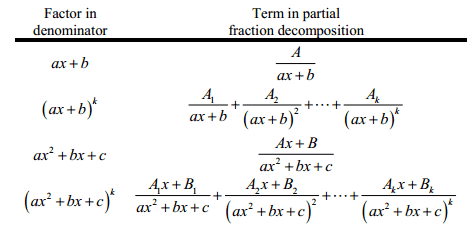# Partial Fraction Decomposition—Multiple Variables

What's the best approach to solving the partial-fraction decomposition of the following expression?

$$\frac{1}{(a-y)(b-y)}$$

The expression is not of the following forms:But I know the solution is

$$= \frac{1}{(a-b)(y-a)}-\frac{1}{(a-b)(y-b)}$$

#### Attachments

ShayanJ
Gold Member
I don't use such tables. Any time I want to do a partial fraction decomposition, I just write (e.g.) $\frac{1}{(a-y)(b-y)}=\frac{A}{a-y}+\frac{B}{b-y}$ and then determine A and B.
Anyway, if you multiply the factors you'll see that its in fact in the form of the third entry in the table!

•Abscissas
Mark44
Mentor
What the table is saying is the for each distinct (i.e., not repeated) factor (ax + b) in the denominator, you'll have a term ##\frac{A}{ax + b}## in the decomposition. So ##\frac{1}{(a - y)(b - y)}## results in ##\frac{A}{a - y} + \frac{B}{b - y}##.

Equate the two expressions and solve for A and B, which is more or less what Shyan said.